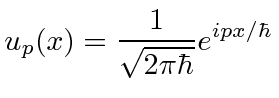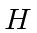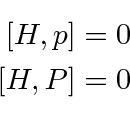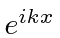## Momentum Eigenfunctions

We can also look at the eigenfunctions of the momentum operator.The eigenstates arewithallowed to be positive or negative.

These solutions do not go to zero at infinity so they are not normalizable to one particle.This is a common problem for this type of state.

We will use a different type of normalization for the momentum eigenstates (and the position eigenstates).Instead of the Kronecker delta, we use the Dirac delta function. The momentum eigenstates have a continuous range of eigenvalues so that they cannot be indexed like the energy eigenstates of a bound system. This means the Kronecker delta could not work anyway.

These are the momentum eigenstatessatisfying the normalization conditionFor a free particle Hamiltonian, both momentum and parity commute with. So we can make simultaneous eigenfunctions.We cannot make eigenfunctions of all three operators sinceSo we have the choice of thestates which are eigenfunctions ofand of, but contain positive and negative parity components. or we have theandstates which contain two momenta but are eigenstates ofand Parity. These are just different linear combinations of the same solutions.

Jim Branson 2013-04-22